# Tournament - Model comparison

This vignette explores the ways you can compare the fit of the different discharge rating curve models provided in the bdrc package. The package includes four different models to fit a discharge rating curve of different complexities. These are:

plm0() - Power-law model with a constant error variance (hence the 0). This is a Bayesian hierarchical implementation of the most commonly used discharge rating curve model in hydrological practice.

plm() - Power-law model with error variance that varies with water elevation.

gplm0() - Generalized power-law model with a constant error variance (hence the 0). The generalized power law is introduced in Hrafnkelsson et al. (2022).

gplm() - Generalized power-law model with error variance that varies with water elevation. The generalized power law is introduced in Hrafnkelsson et al. (2022).

To learn more about the models, see Hrafnkelsson et al. (2022). To learn about how to run the models on your data, see the introduction vignette. The tournament is a model comparison method that uses the Widely Applicable Information Criterion (WAIC) (see Watanabe (2010)) to select the most appropriate of the four models given the data. The WAIC consists of two terms, a measure of the goodness-of-fit, and a penalizing term to account for model complexity (effective number of parameters). The first round of model comparisons sets up two games between model types, “gplm” vs “gplm0” and “plm” vs. “plm0”. The two comparisons are conducted such that if the WAIC of the more complex model (“gplm” and “plm”, respectively) is smaller than the WAIC of the simpler models (“gplm0” and “plm0”, respectively) by a pre-specified value called the winning criteria (default value = 2.2), then it wins the game and is chosen as the more appropriate model. If not, the simpler model is chosen. The more appropriate models move on to the second round and are compared in the same way. The winner of the second round is chosen as the overall tournament winner and deemed the most appropriate model given the data. In each match, the difference in WAIC is defined as $$\Delta$$WAIC$$=$$WAIC$$_{\text{simple}}-$$WAIC$$_{\text{complex}}$$. A positive value of $$\Delta$$WAIC indicates that the more complex model is a more appropriate model, but the more complex model only goes through to the final round if $$\Delta$$WAIC>winning_criteria.

To introduce the tournament function, we will use a dataset from the stream gauging station Krokfors in Sweden that comes with the package:

> library(bdrc)
> data(krokfors)
> krokfors
#>           W          Q
#> 1  9.478000 10.8211700
#> 2  8.698000  1.5010000
#> 3  9.009000  3.3190000
#> 4  8.097000  0.1595700
#> 5  9.104000  4.5462500
#> 6  8.133774  0.2121178
#> 7  8.569583  1.1580000
#> 8  9.139151  4.8110000
#> 9  9.464250 10.9960000
#> 10 8.009214  0.0984130
#> 11 8.961843  2.7847910
#> 12 8.316000  0.6631890
#> 13 8.828716  1.8911800
#> 14 9.897000 20.2600000
#> 15 7.896000  0.0190000
#> 16 9.534000 12.1000000
#> 17 9.114000  4.3560000
#> 18 8.389000  0.6200000
#> 19 8.999000  2.6800000
#> 20 9.099000  3.7310000
#> 21 8.502000  0.8930000
#> 22 8.873000  1.9000000
#> 23 8.240000  0.3200000
#> 24 9.219000  5.9000000
#> 25 9.271000  6.9000000
#> 26 8.370000  0.4420000
#> 27 9.431000  9.0000000

## Running a tournament

The tournament function is easy to use. All you need are two mandatory input arguments, formula and data. The formula is of the form y~x, where y is the discharge in m$$^3/$$s, and x is the water elevation in m (it is very important that the data is in the correct units). The data argument must be a data.frame including x and y as column names. In our case, the dataset from Krokfors has a column named Q which includes the discharge measurements, and a column W which includes the water elevation measurements. We are ready to run our first tournament:

> set.seed(1) # set seed for reproducibility
> t_obj <- tournament(Q~W,krokfors,parallel=TRUE,num_cores=2) # by default parallel=TRUE and the number of cores is detected on the machine
#> Running tournament:
#> 25% - gplm finished
#> 50% - gplm0 finished
#> 75% - plm finished
#> 100% - plm0 finished

The function runs the four models and then the tournament. If you have already run the four different kinds of models, plm0, plm, gplm0 and gplm, and they are stored in objects, say plm0.fit, plm.fit, gplm0.fit and gplm.fit, then you can alternatively run the tournament very efficiently in the following way:

> t_obj <- tournament(list(plm0.fit,plm.fit,gplm0.fit,gplm.fit))

The printing method is very simple and gives you the name of the winner

> t_obj # or alternatively print(t_obj)
#> Tournament with winner gplm0

For a more detailed summary of the results of the tournament, write

> summary(t_obj)
#>   round game model      lppd eff_num_param      WAIC Delta_WAIC winner
#> 1     1    1  gplm  6.320704      6.877144  1.112881  0.5028515  FALSE
#> 2     1    1 gplm0  5.884914      6.692781  1.615733         NA   TRUE
#> 3     1    2   plm -8.903540      4.249257 26.305595 -0.3185198  FALSE
#> 4     1    2  plm0 -8.873488      4.120050 25.987075         NA   TRUE
#> 5     2    3 gplm0  5.884914      6.692781  1.615733 24.3713421   TRUE
#> 6     2    3  plm0 -8.873488      4.120050 25.987075         NA  FALSE

Notice here that in round 1, gplm0 is favored over gplm in the first game, and plm0 over plm in the second. In the second round, gplm0 is deemed the tournament winner, i.e., the model that provides the best simplicity and goodness-of-fit trade-off with the data at hand.

## Comparing different components of the models

There are several tools to visualize the different aspects of the model comparison. To get a visual summary of the results of the different games in the tournament, write

> plot(t_obj) #default plot type is type='tournament_results'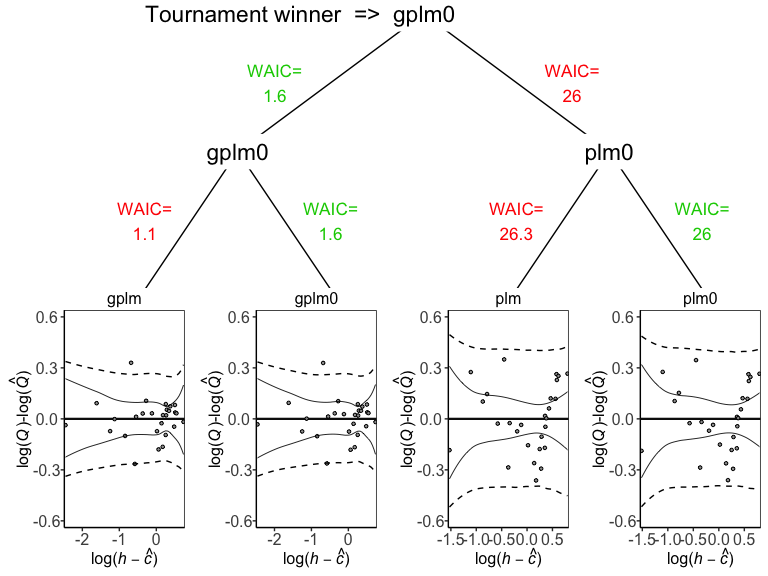An informative way of comparing the goodness-of-fit of the models, is to compare their deviance posteriors. The deviance of an MCMC sample is defined as 2 times the negative log-likelihood of the data given the values of the sampled parameters, therefore, lower values imply a better fit to the data. To plot the posterior distribution of the deviance of the different models, we write

> plot(t_obj,type='deviance')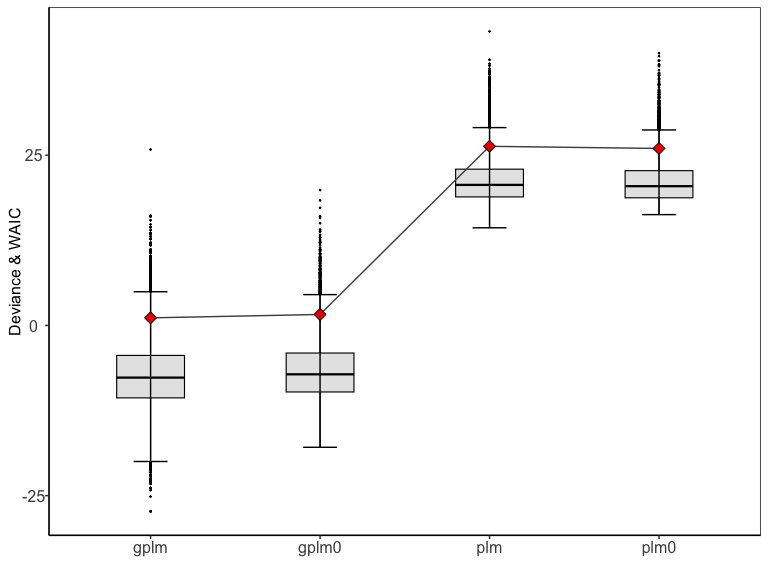The red diamonds on the plot denote the WAIC values for the respective models. Next, to plot the four rating curves that were estimated by the different models, write

> plot(t_obj,type='rating_curve')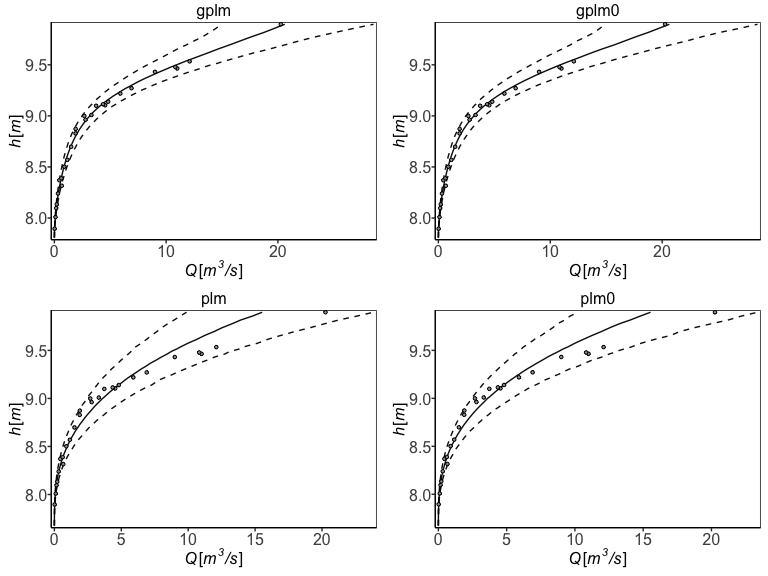Another useful plot is the residual plot

> plot(t_obj,type='residuals')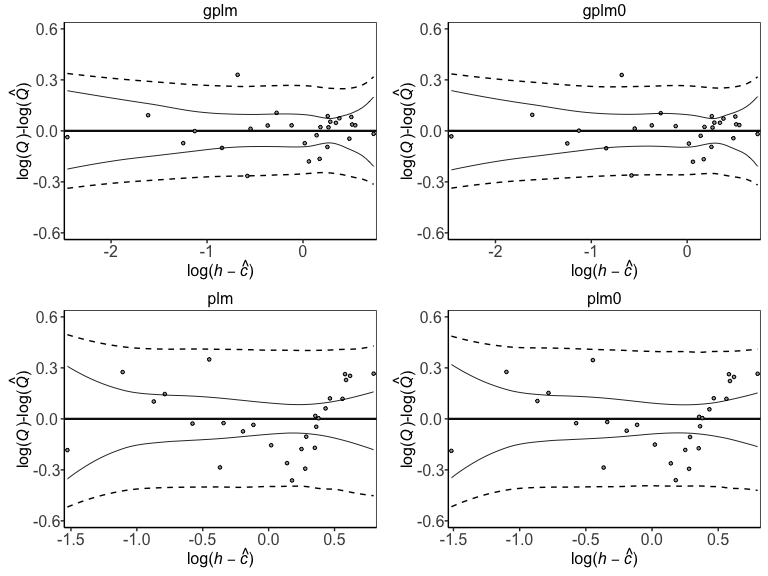The differences between the four models lie in the modeling of the power-law exponent, $$f(h)$$, and the error variance at the response level, $$\sigma^2_{\varepsilon}(h)$$. Thus, it is insightful to look at the posterior of the power-law exponent for the different models

> plot(t_obj,type='f')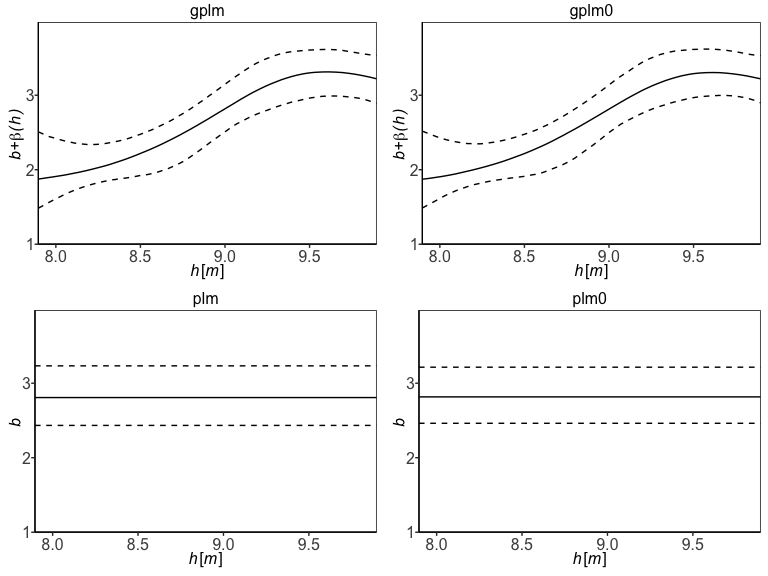and the standard deviation of the error terms at the response level

> plot(t_obj,type='sigma_eps')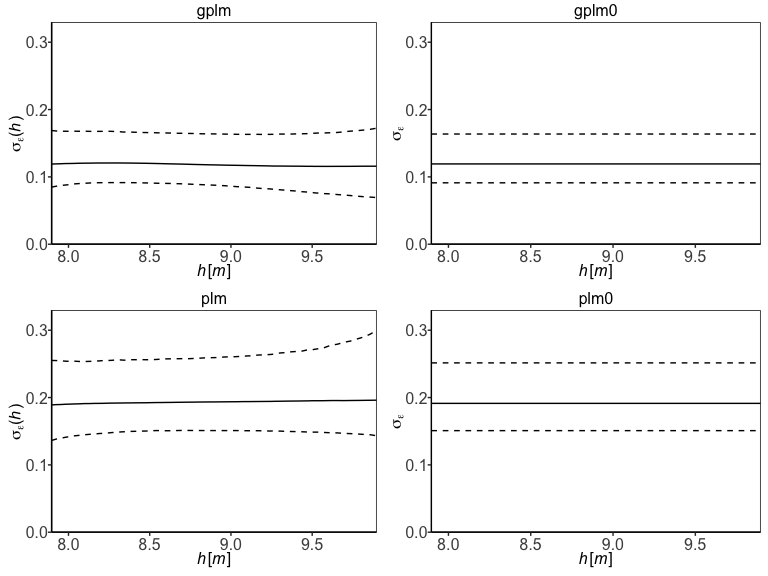Finally, the panel option is useful to gain insight into all different model components of the winning model, which in this case is gplm0:

> plot(t_obj,type='panel',transformed=TRUE)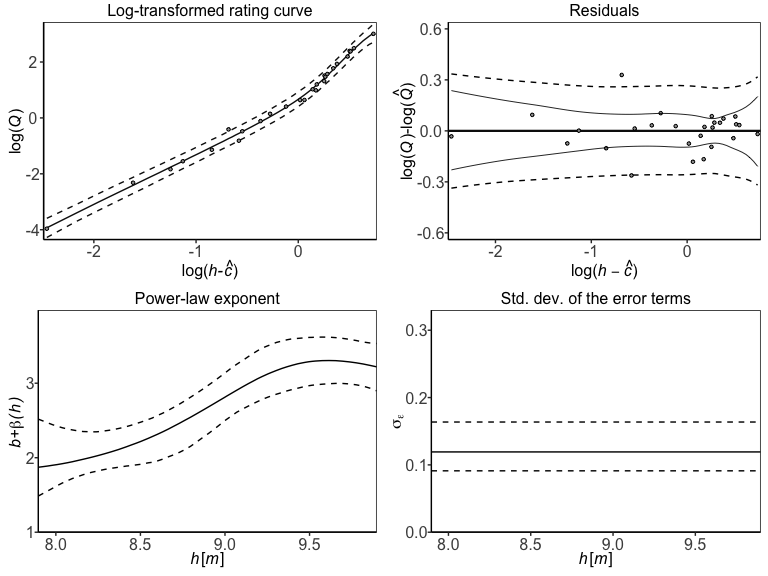## Customizing tournaments

There are a few ways to customize the tournament further. For example, if the parameter of zero discharge $$c$$ is known, you might want to fix that parameter to the known value in the model. Assume 7.65 m is the known value of $$c$$. Then you can directly run a tournament with the $$c$$ parameter fixed in all the models

> t_obj_known_c <- tournament(formula=Q~W,data=krokfors,c_param=7.65)

One can also change the winning criteria (default value = 2.2) which sets the threshold that the more complex model in each model comparison must exceed, in terms of the model comparison criteria (default method is “WAIC”). For example, increasing the value to winning_criteria=5 raises the threshold that the more complex model must exceed to win a game, thus favoring model simplicity more than if the default value of 2.2 were used. To re-evaluate a previously run tournament using a different winning criteria, the most efficient way is to input the list of stored model objects in the existing tournament object. In our case we have the tournament stored in t_obj, so we can write

> t_obj_conservative <- tournament(t_obj$contestants,winning_criteria=5) > summary(t_obj_conservative) #> round game model lppd eff_num_param WAIC Delta_WAIC winner #> 1 1 1 gplm 6.320704 6.877144 1.112881 0.5028515 FALSE #> 2 1 1 gplm0 5.884914 6.692781 1.615733 NA TRUE #> 3 1 2 plm -8.903540 4.249257 26.305595 -0.3185198 FALSE #> 4 1 2 plm0 -8.873488 4.120050 25.987075 NA TRUE #> 5 2 3 gplm0 5.884914 6.692781 1.615733 24.3713421 TRUE #> 6 2 3 plm0 -8.873488 4.120050 25.987075 NA FALSE There is also an option to change the method used to estimate the predictive performance of the models. The default method is “WAIC” (see Watanabe (2010)) which is a fully Bayesian method that uses the full set of posterior draws to calculate the best possible estimate of the expected log pointwise predictive density. Other allowed methods are “DIC” and “Posterior_probability”. The “DIC” (see Spiegelhalter (2002)) is similar to “WAIC” but instead of using the full set of posterior draws to compute the estimate of the expected log pointwise predictive density, it uses a point estimate of the posterior distribution. Both “WAIC” and “DIC” have a default value of 2.2 for the winning criteria. We again run the efficient re-evaluation of the tournament > t_obj_DIC <- tournament(t_obj$contestants,method="DIC")
> summary(t_obj_DIC)
#>   round game model     D_hat eff_num_param        DIC  Delta_DIC winner
#> 1     1    1  gplm -13.85265      6.190845 -1.4709638  0.6520799  FALSE
#> 2     1    1 gplm0 -13.53690      6.359006 -0.8188839         NA   TRUE
#> 3     1    2   plm  17.59492      3.041535 23.6779944 -0.2721095  FALSE
#> 4     1    2  plm0  17.48871      2.958588 23.4058849         NA   TRUE
#> 5     2    3 gplm0 -13.53690      6.359006 -0.8188839 24.2247688   TRUE
#> 6     2    3  plm0  17.48871      2.958588 23.4058849         NA  FALSE

The third and final method that can be chosen is “Posterior_probability”, which uses the posterior probabilities of the models, calculated with Bayes factor (see Jeffreys (1961) and Kass and Raftery (1995)), to compare the models, where all the models are assumed a priori to be equally likely. When using the method “Posterior_probability”, the value of the winning criteria should be a real number between 0 and 1, since this represents the threshold value that the posterior probability of the more complex model has to surpass to be selected as the appropriate model. The default value in this case for the winning criteria is 0.75, which again slightly favors model simplicity. The value 0.75 should give similar results to the other two methods with their respective default values of 2.2. The method “Posterior_probability” is not chosen as the default method because the Bayes factor calculations can be quite unstable. Let’s now use this method, but raise the winning criteria from 0.75 to 0.9

> t_obj_prob <- tournament(t_obj\$contestants,method="Posterior_probability",winning_criteria=0.9)
> summary(t_obj_prob)
#>   round game model     marg_lik    Post_prob winner
#> 1     1    1  gplm 3.201743e-02 1.302100e-01  FALSE
#> 2     1    1 gplm0 2.138732e-01 8.697900e-01   TRUE
#> 3     1    2   plm 1.427185e-06 4.339125e-01  FALSE
#> 4     1    2  plm0 1.861923e-06 5.660875e-01   TRUE
#> 5     2    3 gplm0 2.138732e-01 9.999913e-01   TRUE
#> 6     2    3  plm0 1.861923e-06 8.705656e-06  FALSE

We see that the results of the tournament do not change in this example, and the winner of the third and final game is still gplm0.